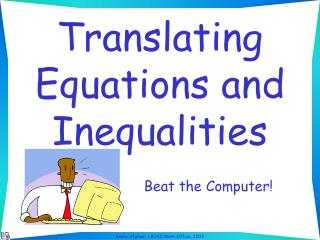DownloadDownload PresentationTranslating Equations and Inequalities

Translating Equations and Inequalities

Download PresentationTranslating Equations and Inequalities

- - - - - - - - - - - - - - - - - - - - - - - - - - - E N D - - - - - - - - - - - - - - - - - - - - - - - - - - -
Presentation Transcript

1. Translating Equations and Inequalities Beat the Computer!

2. Directions: • Say the equation or inequality out loud before the computer gives you the answer • Use n for the variable • The slides will advance automatically

3. The difference of eleven and a number is five

4. Twenty less than a number is three

5. Twenty less than a number is three n - 20 = 3

6. Twelve more than a number is sixteen

7. Twelve more than a number is sixteen 12 + n = 16

8. The product of four and a number, minus three is seventeen

9. The quotient of a number and five is greater than two

10. Six divided by a number is less than or equal to five

11. The sum of six and number is at least two

12. Six multiplied by a number, take away five is less than seven 6n - 5 < 7

13. The sum of six and number all divided by three is two

14. Five less than twice a number is seven

15. Five less than twice a number is seven 2n - 5 = 7